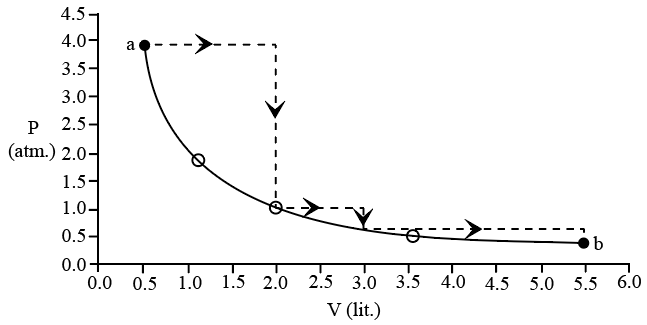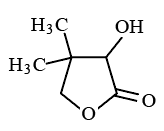Instructions

For the following questions answer them individually

Question 11

# One mole of an ideal gas is taken from a to b along two paths denoted bythe solid and the dashed lines as shownin the graph below. If the work done along the solid line path is $$W_s$$ and that along the dotted line path is $$W_d$$, then the integer closest to the ratio $$\frac{W_d}{W_s}$$ isBackspace
789
456
123
0.-
Clear All
Instructions

Two aliphatic aldehydes P and Q react in the presence of aqueous $$K_2CO_3$$ to give compound R, which upon treatment with HCN provides compound S. On acidification and heating, S gives the product shown below:Question 12

Question 13

Question 14

# The compound S is

Instructions

The hydrogen-like species $$Li^{2+}$$ is in a spherically symmetric state $$S_1$$, with one radial node. Upon absorbing light the ion undergoes transition to a state $$S_2$$. The state $$S_2$$, has one radial node and its energy is equal to the groundstate energy of the hydrogen atom.

Question 15

Question 16

Question 17

# The orbital angular momentum quantum number of the state $$S_2$$ is

Instructions

For the following questions answer them individually

Question 18

Backspace
789
456
123
0.-
Clear All
Question 19

Backspace
789
456
123
0.-
Clear All
Question 20

OR